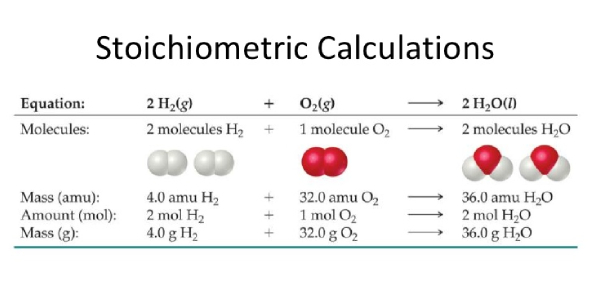Stoichiometry Calculating Quiz! Trivia

3 Questions | Total Attempts: 313Settings.

• 1.
If you have 4.5 moles of lead(IV) nitrate how many moles of silver nitrate can be produced according to the following reaction? Pb(NO3)4 + 4AgCl ---> 4AgNO3 + PbCl4
• A.

1.2n

• B.

4.5n

• C.

16n

• D.

18n

• 2.
Starting with 31g of Al in the following reaction will produce hao many grams of Na? Na3PO4 + Al ---> AlPO4 + 3Na
• A.

8.8g

• B.

9.0g

• C.

79g

• D.

79.2g

• 3.
If you counted 6.0 x 10^24 molecules of hydrogen gas how many molecules of oxygen gas will be need to completely react the amount according to the following equation? 2H2 + O2 ---> 2H2O
• A.

3.0 x 10^23

• B.

1.2 x 10^24

• C.

3.0 x 10^24

• D.

1.2 x 10^25

Related TopicsBack to top